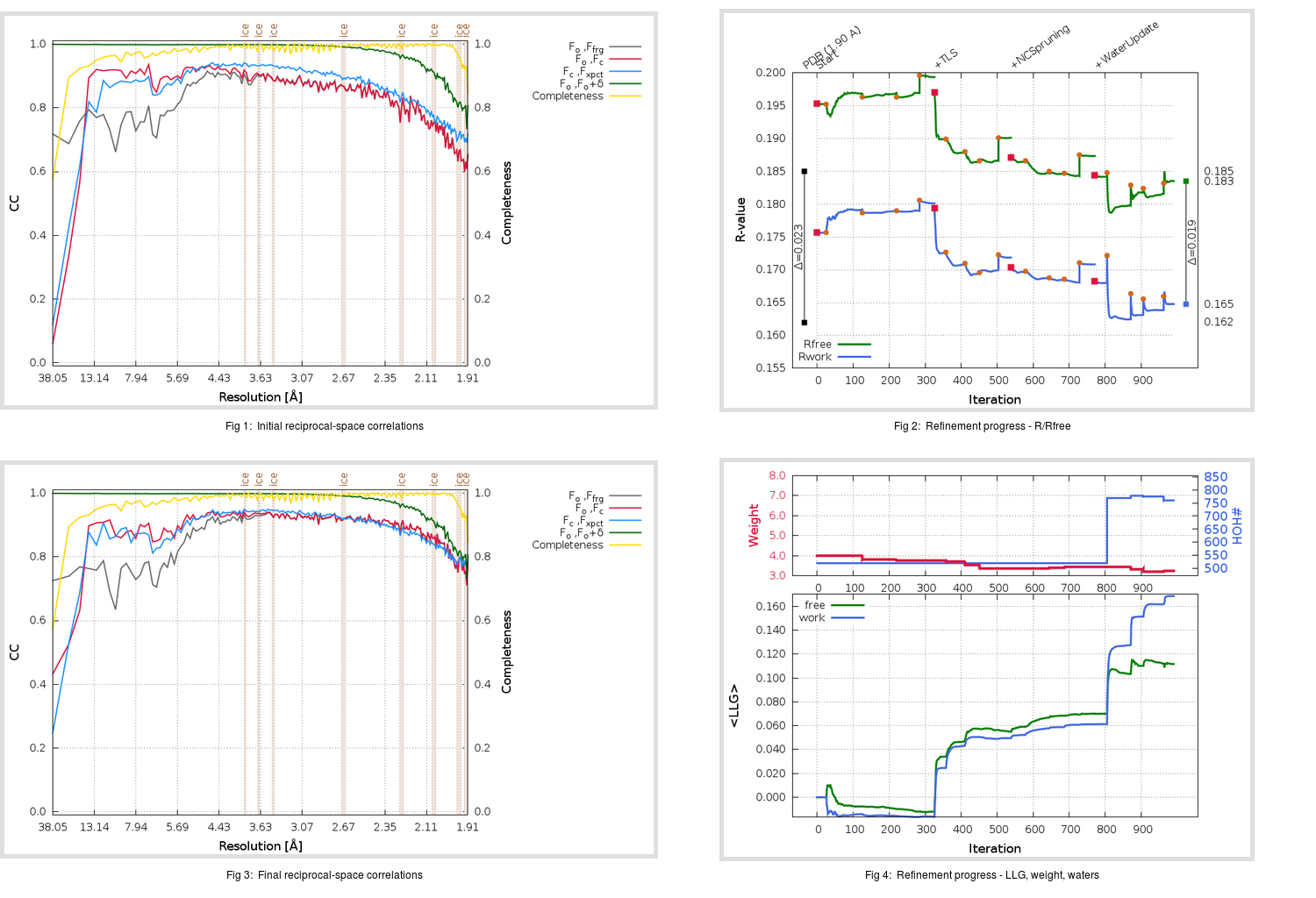Content:

```    Diffraction limits & principal axes of ellipsoid fitted to diffraction cut-off surface:
1.884         1.0000   0.0000   0.0000       0.894 a* - 0.447 b*
1.884         0.0000   1.0000   0.0000       b*
1.895         0.0000   0.0000   1.0000       c*
```

## Deposited

` `
 Date deposited Date data collection Resolution R, Rfree 20200228 20200225 1.90 0.1610 0.1850

Molprobity (CCP4 7.0 version) summary:

```Ramachandran outliers =   0.00 %
favored =  98.26 %
Rotamer outliers      =   0.95 %
C-beta deviations     =     0
Clashscore            =   2.17
RMS(bonds)            =   0.0117
RMS(angles)           =   1.30
MolProbity score      =   0.99
Resolution            =   1.90
R-work                =   0.1610
R-free                =   0.1850
```

```Number of waters      =   519

<B> (all atoms) =   41.25 ( sd =   14.68 ) for       6253 non-hydrogen atoms
<B>   (protein) =   40.33 ( sd =   14.51 ) for       5599 non-hydrogen atoms
<B>     (water) =   45.41 ( sd =   10.65 ) for        519 non-hydrogen atoms
<B>    (others) =   62.60 ( sd =   15.21 ) for        135 non-hydrogen atoms

B min/max       (all non-hydrogen atoms) =   19.71 /  111.79
B min/max   (protein non-hydrogen atoms) =   19.71 /  111.79
B min/max     (water non-hydrogen atoms) =   23.71 /   70.18
B min/max     (other non-hydrogen atoms) =   30.87 /   94.54
```

## BUSTER (re-)refinement

` `

Molprobity (CCP4 7.0 version) summary:

```Ramachandran outliers =   0.00 %
favored =  98.55 %
Rotamer outliers      =   1.11 %
C-beta deviations     =     1
Clashscore            =   2.09
RMS(bonds)            =   0.0120
RMS(angles)           =   1.52
MolProbity score      =   1.01
Resolution            =   1.90
R-work                =   0.1648
R-free                =   0.1835
```

```Number of waters      =   760

<B> (all atoms) =   40.77 ( sd =   13.84 ) for       6494 non-hydrogen atoms
<B>   (protein) =   38.61 ( sd =   12.31 ) for       5599 non-hydrogen atoms
<B>     (water) =   52.95 ( sd =   15.04 ) for        760 non-hydrogen atoms
<B>    (others) =   59.97 ( sd =   12.86 ) for        135 non-hydrogen atoms

B min/max       (all non-hydrogen atoms) =   20.76 /   96.36
B min/max   (protein non-hydrogen atoms) =   20.76 /   87.46
B min/max     (water non-hydrogen atoms) =   22.09 /   96.36
B min/max     (other non-hydrogen atoms) =   36.37 /   84.10
```

Refinement progression:Results:

` `
 File Remark 6W01_aB_refine.01_04_refine.pdb.gz exact refinement commands are in header 6W01_aB_refine.01_04_refine.mtz.gz including original deposited data and several re-refinement map coefficients 6W01_aB_refine.01_04_BUSTER_model.cif.gz including any non-standard compound restraints 6W01_aB_refine.01_04_BUSTER_refln.cif.gz